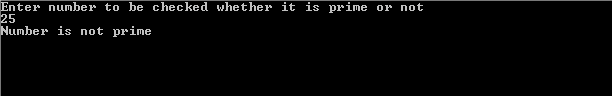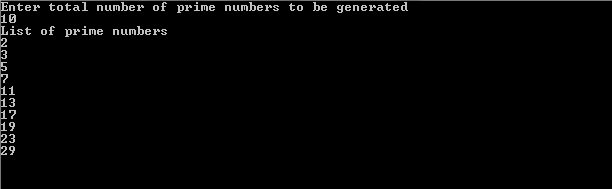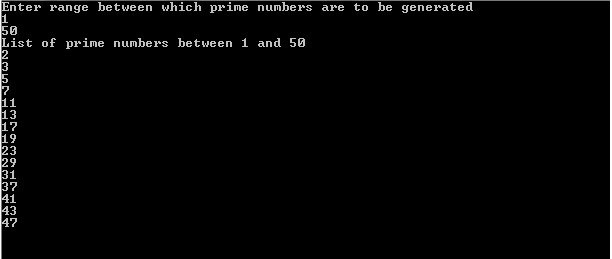# Prime Number Program in C – C Program for Prime Number

Prime number program in c along with the output is given below.

## About Prime Numbers

Any natural number like 1, 2, 3, etc. is called a prime or a prime number if it is greater than 1 and can only be divided by 1 and the number itself.

For example,
2, 3, 5, 7, 11, 13, 17, 19, 23, 29,…, and so on

It should be noted that the natural numbers greater than 1 that are not prime numbers are called composite.

## Prime Number Program in C – Program 1

The below c program for prime number takes an integer as input, and checks whether the input number is a prime number or not.

```#include<stdio.h>
void main()
{
int x,i,flag=0;
printf("Enter number to be checked whether it is prime or notn");
scanf("%d",&x);
if(x>0)
{
for(i=2; i<=x/2; i++)
{
if(x%i==0)
{
flag=1;
break;
}
}
if(flag==0 && x!=1)
printf("Number is primen");
else
printf("Number is not primen");
}
else
printf("Please enter number greater than 0n");

getch();
}
```

### Output## Prime Number Program in C – Program 2

The below c program for prime number prints the total number of prime numbers provided as an input by the user.

```#include<stdio.h>

void main()
{
int n,count=1,i,flag=0;
printf("Enter total number of prime numbers to be generatedn");
scanf("%d",&n);
printf("List of prime numbersn");
for(i=2; count<=n; i++)
{
flag=prime(i);
if(flag==1)
{
printf("%dn",i);
count++;

}
}
getch();
}
int prime(int x)
{
int j;
for(j=2; j<=x/2; j++)
{
if(x%j==0)
{
return 0;
break;
}

}
if(j>x/2)
return 1;
}
```## Prime Number Program in C – Program 3

The below c program for prime number prints all the prime numbers between a range as mentioned by the user.

```#include<stdio.h>

void main()
{
int start,end,i,flag=0;
printf("Enter range between which prime numbers are to be generatedn");
scanf("%d%d",&start,&end);
printf("List of prime numbers between %d and %dn",start,end);
for(i=start; i<=end; i++)
{
flag=prime(i);
if(flag==1 && i!=1)
printf("%dn",i);
}
getch();
}
int prime(int x)
{
int j;
for(j=2; j<=x/2; j++)
{
if(x%j==0)
{
return 0;
break;
}

}
if(j>x/2)
return 1;
}
```

### Output### 2 Responses

1.sowji at | | Reply

program input give little more easy .programs which are give are difficult to understand.

Please comment with your real name using good manners.

This site uses Akismet to reduce spam. Learn how your comment data is processed.